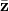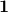Warning: "continue" targeting switch is equivalent to "break". Did you mean to use "continue 2"? in /home/calcul9/public_html/wp-content/themes/suffusion/functions/media.php on line 580

Warning: "continue" targeting switch is equivalent to "break". Did you mean to use "continue 2"? in /home/calcul9/public_html/wp-content/themes/suffusion/functions/media.php on line 583

Warning: "continue" targeting switch is equivalent to "break". Did you mean to use "continue 2"? in /home/calcul9/public_html/wp-content/themes/suffusion/functions/media.php on line 586

Warning: "continue" targeting switch is equivalent to "break". Did you mean to use "continue 2"? in /home/calcul9/public_html/wp-content/themes/suffusion/functions/media.php on line 589

Warning: "continue" targeting switch is equivalent to "break". Did you mean to use "continue 2"? in /home/calcul9/public_html/wp-content/themes/suffusion/functions/media.php on line 592
2011 June 07 » The Calculating Investor

## Calculating the Efficient Frontier: Part 1

Jun 072011
###### A Matrix Based Example of  Mean-Variance Optimization using Octave

The concept of  an “efficient frontier” was developed by Harry Markowitz in the 1950s.  The efficient frontier shows us the minimum risk (i.e. standard deviation) that can be achieved at each level of expected return for a given set of risky securities.

Of course, to calculate the efficient frontier, we need to have an estimate of the expected returns and the covariance matrix for the set of risky securities which will used to build the optimal portfolio.  These parameters are difficult (impossible) to forecast, and the optimal portfolio calculation is extremely sensitive to these parameters.  For this reason, an efficient frontier based portfolio is difficult to successfully implement in practice.  However, a familiarity with the concept is still very useful and will help to develop intuition about diversification and the relationship between risk and return.

###### Calculating the Efficient Frontier

In this post, I’ll demonstrate how to calculate and plot the efficient frontier using the expected returns and covariance matrix for a set of securities.

In a future post, I’ll demonstrate how to calculate the security weights for various points on this efficient frontier using the two-fund separation theorem.

###### Calculations

In order to calculate the efficient frontier using n assets, we need two inputs.  First, we need the expected returns of each asset.  The vector of expected returns will be designated.  The second input is the variance-covariance matrix for the n assets.  This covariance matrix will be designated as.  We also need a unity vector () with the same length as the vector.

Once we have this information, we can run the following calculations using a matrix based mathematical program such as Octave or Matlab.